•5星
599KB GZXGYZ 2021-04-12 16:39:55
• 376KB weixin_38710323 2021-05-25 23:54:04
• 三次函数的对称中心问题 数学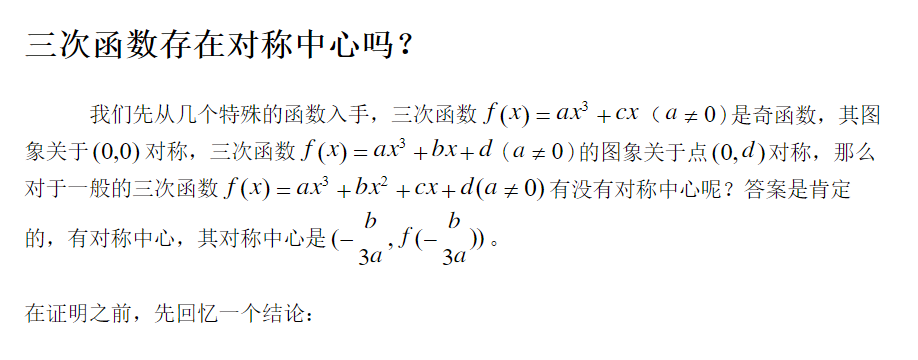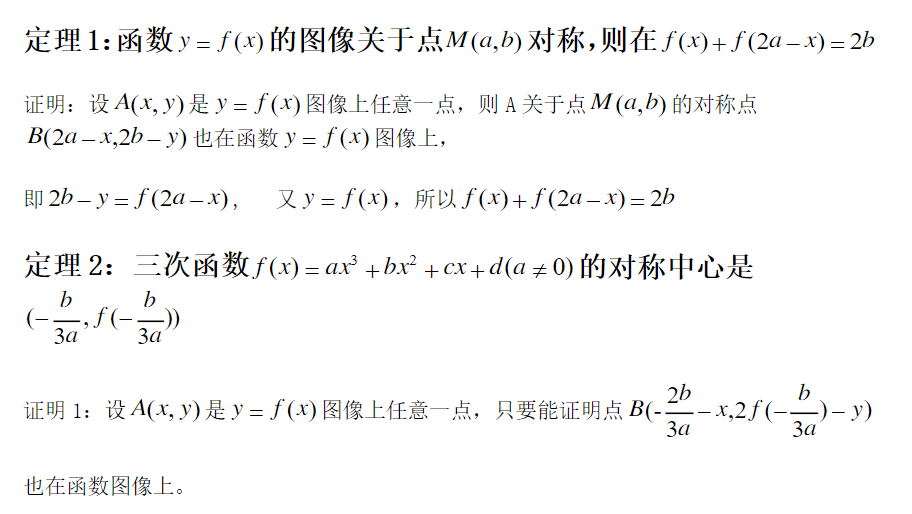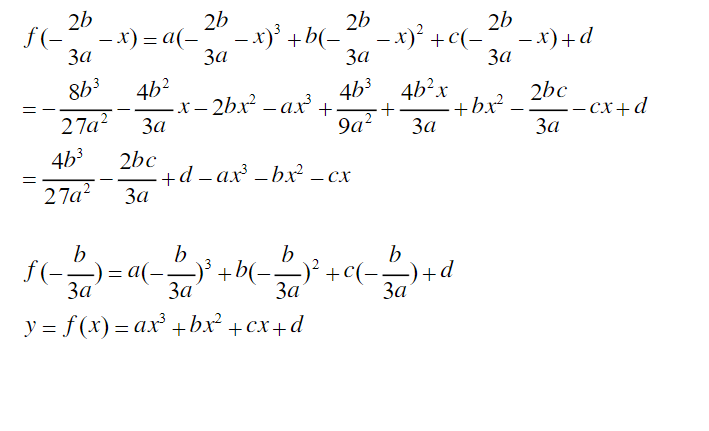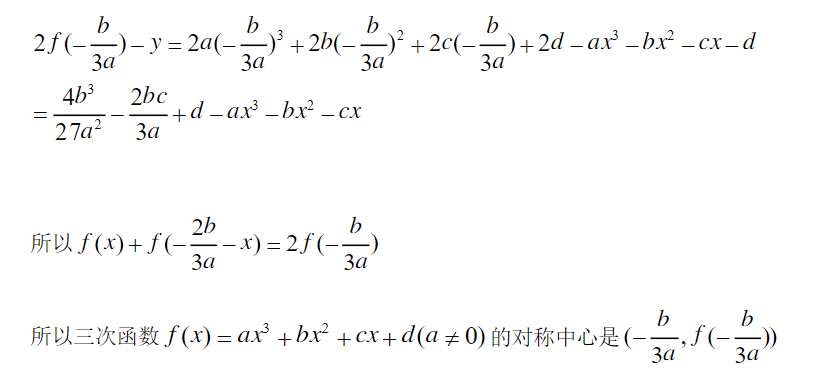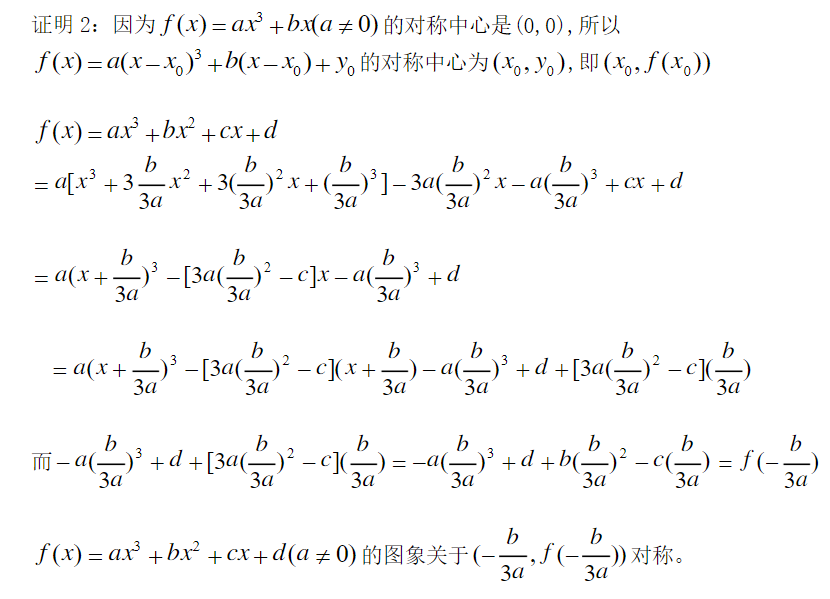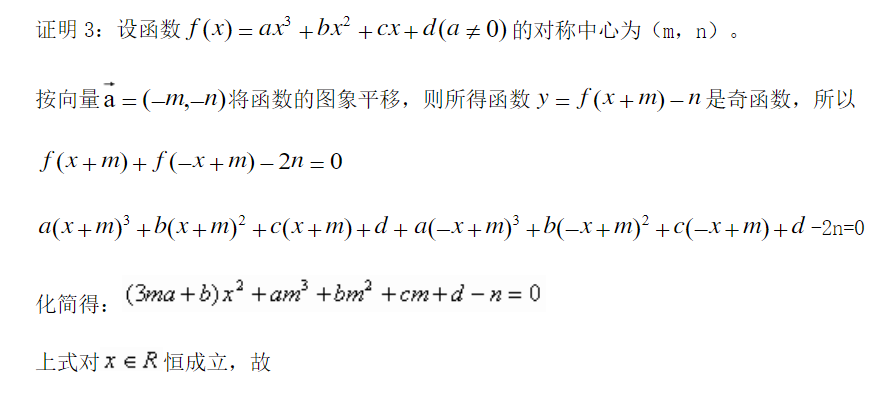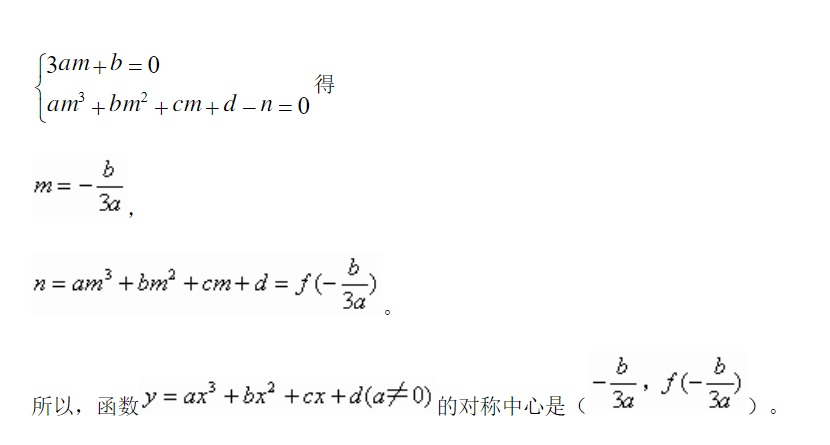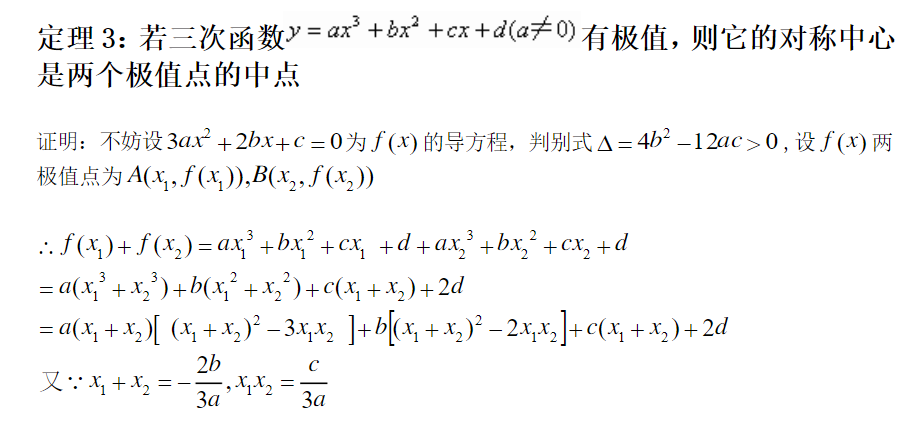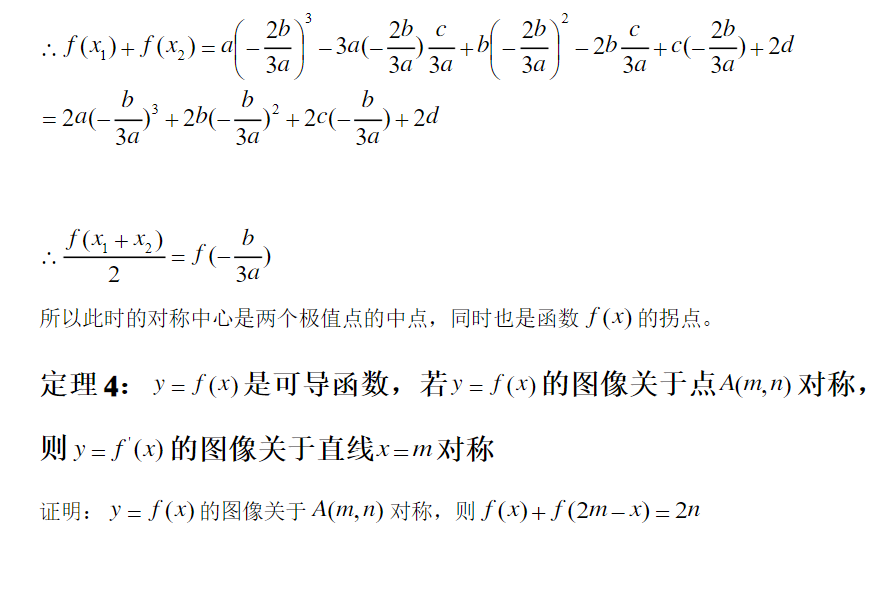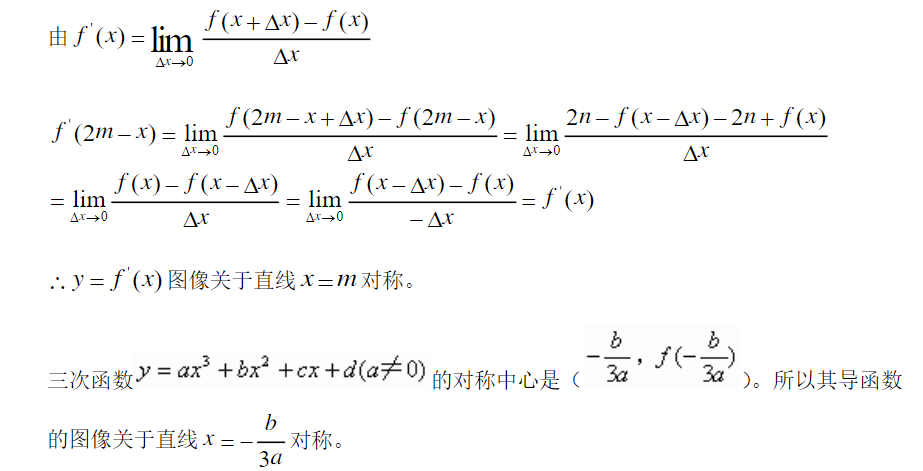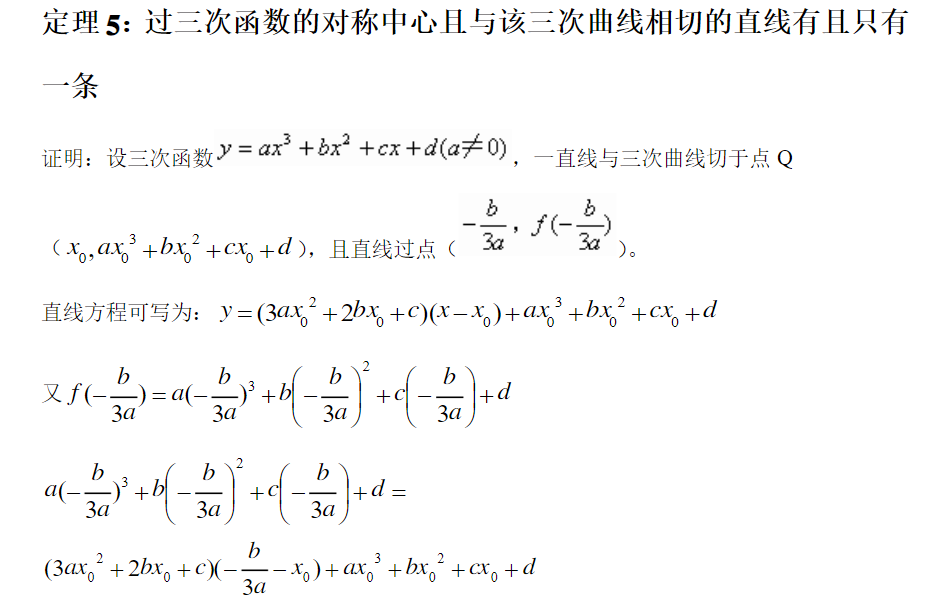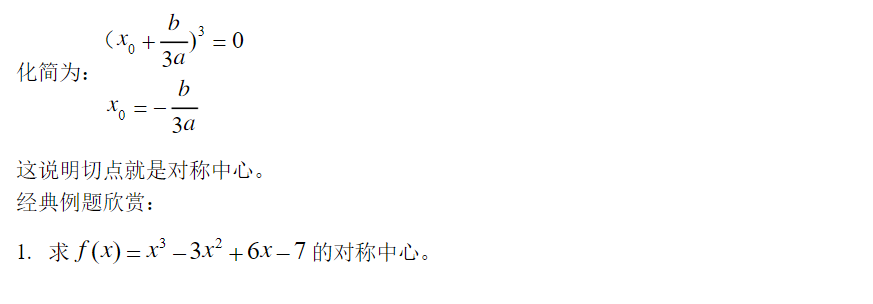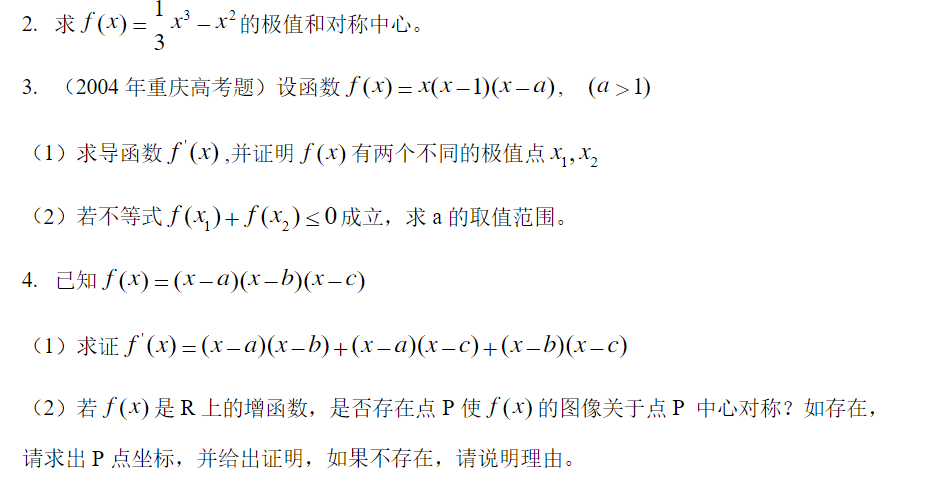展开全文zsjzliziyang 2020-10-20 21:16:24
• # 背景#### 材料力学老师给布置了一个很坑的核心的问题，花了我一个多小时才做出来......于是有了编程解决此类问题的想法。----------# 说明#### 由于还没有开发完全，本程序支持的横截面至少有一个与坐标轴垂直的...

# 背景

#### 材料力学老师给布置了一个很坑的求核心的问题，花了我一个多小时才做出来......于是有了编程解决此类问题的想法。

----------

# 说明

#### 由于还没有开发完全，本程序支持的横截面至少有一个与坐标轴垂直的对称轴

----------

# 测试

## 测试1

#### ![](https://leanote.com/api/file/getImage?fileId=58fb0a15ab644129eb004014)

![](https://leanote.com/api/file/getImage?fileId=58fb0a15ab644129eb004016)

## 测试2

#### ![](https://leanote.com/api/file/getImage?fileId=58fb0a15ab644129eb004017)

![](https://leanote.com/api/file/getImage?fileId=58fb0a15ab644129eb004015)

## 测试3

### 注：

#### 这里由于内部结构过于复杂，目前还没有做到可以使用线的形式来表示，所以只能先用点簇形式表示，最后人工连线。

#### ![](https://leanote.com/api/file/getImage?fileId=58fb0a15ab644129eb004018)

![](https://leanote.com/api/file/getImage?fileId=58fb0a15ab644129eb004019)

## 测试4

####

![](https://leanote.com/api/file/getImage?fileId=58fb0d52ab644129eb004072)

![](https://leanote.com/api/file/getImage?fileId=58fb0d52ab644129eb004073)

### 注：

#### 这里有图像失真，这可能是由于计算机在处理较大整数的时候有舍入误差导致的，由于也不是计算机专业的，暂时不知道如何优化。

----------

# 原理

####

1. 使用图像识别出某个黑白图片的区域和边缘，对边缘进行矢量化，进行拟合后得到数据

2. 按照材料力学原理构建模型进行绘图(这里假设每一个像素为一个基本面元)

----------

# 理论支持

####

- 求形心

$${S_x} = \int\int\limits_\Omega {ydxdy}$$

$${S_x} = {X_c} \times A$$

可由此求出${X_c}$的值

同理可以求出${Y_c}$的值

####

- 进行坐标变换，将原本图像的坐标轴变换至新的形心坐标轴内

####

- 由

$${i_x}^2 \times A = \int\int {{y^2}dxdy}$$

$${i_y}^2 \times A = \int\int {{x^2}dxdy}$$

可以求得${i_x}^2$、${i_y}^2$

####

- 由

$${a_x} = - {{{i_y}^2} \over {{X_F}}}$$

$${a_y} = - {{{i_x}^2} \over {{Y_F}}}$$

得到${X_F}$、${Y_F}$的值

####

- 迭代；作图

----------

# 代码

## In "CoreFunction.m"

####

function CoreFunction(ImageLocation,M,N)

%% Image initial

imshow(Image);

title('OriginImage');

hold off;

Temp = input('This is the original image.\nPress any key to continue.\n\n');

TwoBitImage = im2bw(Image);

TwoBitImage = imresize(TwoBitImage,[400 400]);

% black in 0;white in 1

[X Y] = find(~TwoBitImage);

DotInImage = [X Y];

% Dots in black area

imshow(TwoBitImage);

title('ImageInBlackAndWhite');

Temp = input('This is the two-bit form.\nPress any key to continue.\n\n');

EdgeImage = edge(TwoBitImage,'canny');

% Edge in black area

% black in 0;white in 1;

imshow(EdgeImage);

title('EdgeImage');

[X,Y]=find(EdgeImage);

EdgeData =[X Y];

Temp = input('This is the Edge form.\nPress any key to continue.\n\n');

%% Edge data sort

EdgeData = SortData(EdgeData(2:end,:),[],X(1),Y(1));

%% Check

X = EdgeData(:,1);

Y = EdgeData(:,2);

plot(Y,X);

%% Filter

EdgeData = EdgeData(1:M:end,:);

X = EdgeData(:,1);

Y = EdgeData(:,2);

plot(Y,X);

hold on

%% Confirm Poid(XingXin)

% Coordinate of Xc = Sy / A;

Sy = sum(DotInImage(:,2));

A = length(DotInImage);

Xc = (Sy / A);

% Coordinate of Yc = Sx / A;

Sx = sum(DotInImage(:,1));

Yc = (Sx / A);

% Scatter

scatter(Yc,Xc)

% using Parallel Axis Fomula

% Ix = Ixc + a^2*A

%Ixc = sum(DotInImage(:,2).^2) - Yc^2*A;

Ixc = sum((DotInImage(:,2)-Yc).^2);

ix_2 = (Ixc/A);

% Iy = Iyc + b^2*A

%Iyc = sum(DotInImage(:,1).^2) - Xc^2*A;

Iyc = sum((DotInImage(:,1)-Xc).^2);

iy_2 = (Iyc/A);

%% Confirm ax and ay(QiuGeGeDianDeQieXianZaiXinXingZhuZhouDeJieJu)

InterceptDataInXcYc = Intercept(Xc,Yc,X(1:end-2),Y(1:end-2),X(3:end) ...

,Y(3:end));

ax = InterceptDataInXcYc(:,1);

ay = InterceptDataInXcYc(:,2);

%% Calculate Xf and Yf

Xf = -iy_2./ax;

Yf = -ix_2./ay;

%% Inverse Coordinate Transformation

Xf = (Xf + Xc);

Yf = (Yf + Yc);

R = SortData([Xf Yf],[],Xf(1),Yf(1));

% N

%

Xf = R(1:N:end,1);

Yf = R(1:N:end,2);

% Filter

Dic = (Xf<0|Xf>400);

Xf(Dic)=[];

Yf(Dic)=[];

Dic = (Yf<0|Yf>400);

Xf(Dic)=[];

Yf(Dic)=[];

% Catinate

Xf = [Xf;Xf(1)];

Yf = [Yf;Yf(1)];

scatter(Yf,Xf,'.','k');

set(gca,'ydir','reverse')

end

## In "Intercept.m"

####

function Output = Intercept(Xc,Yc,X0,Y0,X1,Y1)

%% Initial

%% Tranform

X0 = X0 - Xc;

Y0 = Y0 - Yc;

X1 = X1 - Xc;

Y1 = Y1 - Yc;

%% Calculate

K = (Y1-Y0)./(X1-X0);

X = X0 - Y0./K;

Y = -K.*X0 + Y0;

%%

Output = [X Y];

Output = SortData(Output(2:end,:),[],Output(1,1),Output(1,2));

end

## In "SortData.m"

####

function R = SortData(OldData,NewData,X0,Y0)

%% Initial

X = OldData(:,1);

Y = OldData(:,2);

%% Distance

Distance = sqrt((X0-X).^2+(Y0-Y).^2);

OldData = [X Y Distance];

%% Sort and append

OldData = sortrows(OldData,3);

X0 = OldData(1,1);

Y0 = OldData(1,2);

OldData = OldData(2:end,[1 2]);

NewData = [NewData;[X0 Y0]];

%% Recursion

if(length(OldData) > 0)

R = [SortData(OldData,NewData,X0,Y0);[X0 Y0]];

else

R = [];

end

end

----------

# 待解决问题

- 在求解尖角处的数据时会有瑕疵

- 计算机会溢出很大的整数

- 由于知识受限，只能求解至少有一个对称轴的截面，不知道如果两个形心主轴不垂直应该如何去解决求核心问题

- 截面有孔的情况不知道怎么处理

展开全文weixin_42514107 2021-04-18 12:05:21
• 在机器学习中，以零为中心的协方差矩阵就是这种形式。 如果 A的列是线性无关的，则 AᵀA是可逆的。 每个对称矩阵小号可以进行对角化(因式分解)与Q由正交的特征向量形成vᵢ的小号和Λ是对角矩阵保持所有的特征值。 每...在线性代数中，有一些特殊的矩阵具有易于分析和操作的特性。它们的特征向量可能具有特定的特征值或特殊关系。还有一些方法可以将一个矩阵分解成这些“更简单”的矩阵。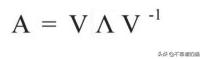操作复杂性的降低提高了可伸缩性。然而，即使这些矩阵都是特殊的，它们也不是罕见的。在机器学习和许多应用程序中，我们经常需要处理它们。

对角矩阵

对角矩阵S使所有非对角元素等于零。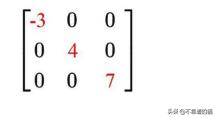许多分解方法都有一个分解后的矩阵是对角矩阵。由于矩阵只包含对角元素，我们有时用向量来表示它。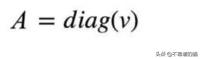一般矩阵的逆不容易计算。但是求对角矩阵的逆很简单。我们可以用1/m替换对角线元素。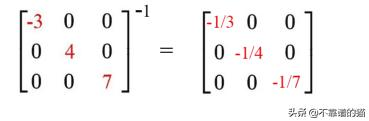如果其中一个矩阵是对角的，矩阵乘法就简单多了。但是当任何对角元素等于0或者对角矩阵不是方形的时候，它的逆就不存在。但是，在一些方法中，伪逆矩阵(keep the inverse of 0 as 0)可以用作替代。

正交矩阵

正交矩阵Q是满足下列要求的方形矩阵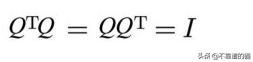Q中的所有列(v 1 ，...，v 6 ，...)都是正交的，即对于i≠j，vᵢᵀvⱼ= 0，vᵢ都是单位向量。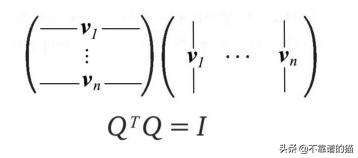这听起来像是一个严格的要求但是对于一些矩阵，比如对称矩阵，我们可以选择特征向量在分解过程中是正交的。

以下矩阵是正交的。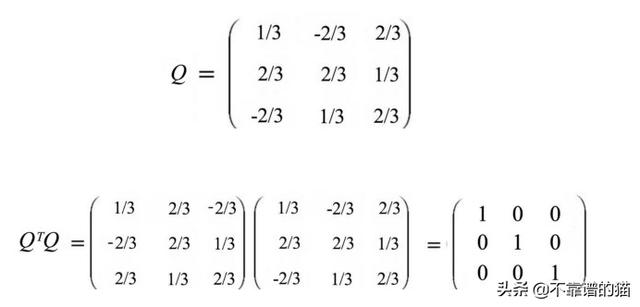像对角矩阵一样，它的逆也很容易计算 - 正交矩阵的逆是它的转置。这是正交矩阵非常方便的一个关键原因。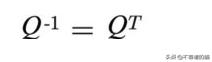证明：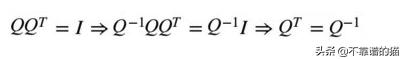如果我们用正交矩阵乘以x, x中的误差不会被放大。这种行为对于保持数值稳定性是非常理想的。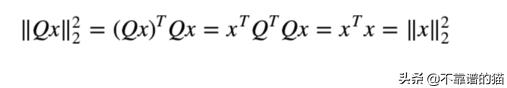对称矩阵

如果矩阵的转置等于自身，则矩阵是对称的。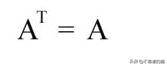例如，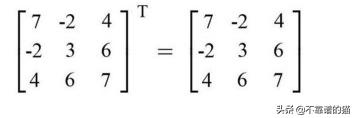对称矩阵是线性代数和机器学习中最重要的矩阵之一。在机器学习(ML),我们经常使用矩阵保存f(vᵢ , vⱼ)。这些函数通常是对称的，f(x, y) = f(y, x)，因此对应的矩阵是对称的。例如在机器学习中，f可以测量数据点之间的特征距离，或者计算特征的协方差。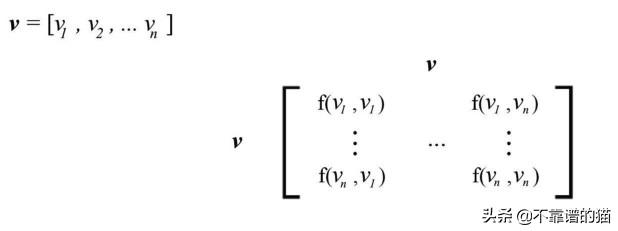对称矩阵属性

对称矩阵S是n×n方形矩阵。

• 它的逆也是对称的。
• S的所有特征值都是实数(不是复数)。
• 即使重复的特征值，我们也可以选择S的 n个本征向量为正交。
• 可以通过将矩阵A与其转置 - AᵀA或AAᵀ(通常AᵀA ≠ AAᵀ)相乘来形成对称矩阵。在机器学习中，以零为中心的协方差矩阵就是这种形式。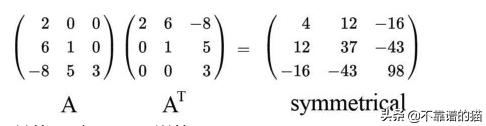• 如果 A的列是线性无关的，则 AᵀA是可逆的。
• 每个对称矩阵小号可以进行对角化(因式分解)与Q由正交的特征向量形成vᵢ的小号和Λ是对角矩阵保持所有的特征值。
• 每个对称矩阵S可以被对角化(分解)，其中Q由S的正交特征向量vi形成，Λ是对角矩阵的所有特征值。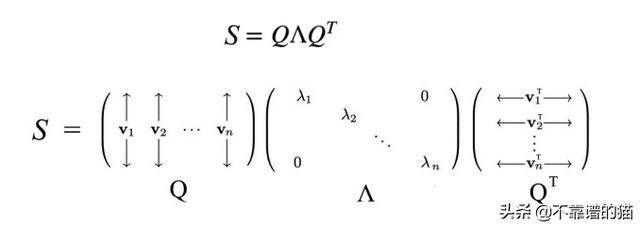上面的等式可以改写为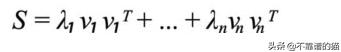其中v是单位向量。因此，特征值项λᵢ主导了上述每个项的重要性。事实上，如果它太小，我们可以完全放弃相应的项λᵢvᵢvᵢᵀ。

该分解特性和“ S具有n个正交特征向量”是对称矩阵的两个重要特性。

正交特征向量

特征向量不是唯一的。但通常，我们可以“选择”一组特征向量来满足某些特定条件。如前所述，对称矩阵的特征向量可以选择为正交。如果S是对称矩阵，则其特征值λ和μ满足以下条件。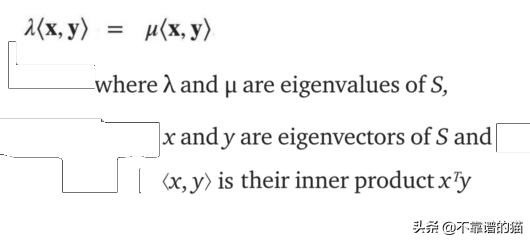证明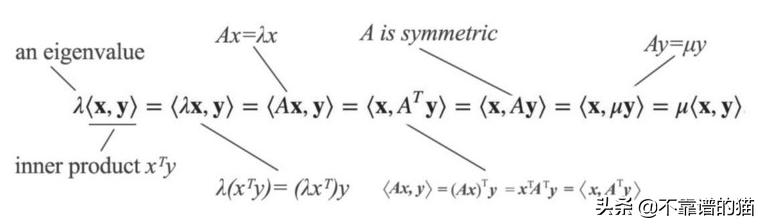从这种情况来看，如果λ和μ具有不同的值，则等效性迫使内积为零。因此，x和y是正交的，并且很容易将它们归一化为具有单位长度 - 正交。这证明了如果它们的相应特征值不同，我们可以选择S的特征向量是正交的。即使有重复的特征值，对于对称矩阵仍然如此。

证明 - 第2部分(可选)

对于n×n对称矩阵，我们总能找到n个独立的正交特征向量。最大的特征值是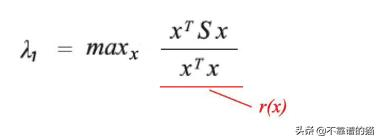为了求最大值，我们令r(x)的导数为0。经过一些处理，得到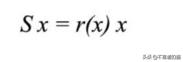即，当x是特征向量且特征值最大时，r(x)的比值最大。通过归纳，我们可以推导出我们可以用正交于前一个的特征向量找到下一个最高的特征值。这只是证明的高级描述。

谱定理(Spectral theorem)

让我们总结一下。每个n×n对称矩阵S具有n个实特征值λᵢ，其中有n个正交特征向量vᵢ。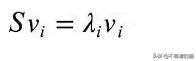这些特征值可以形成对角矩阵Λ as diag(λ)。我们还可以将特征向量vᵢ连接到V，即，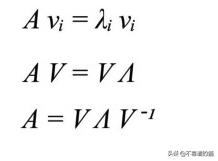我们将V重命名为Q.因为Q是正交的，所以它是可逆的并且Qᵀ= Q-1。因此，对称矩阵S可以分解为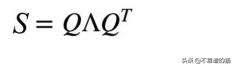这是谱定理。因为找到转置比逆转更容易，所以在线性代数中非常需要对称矩阵。

正定矩阵

正定矩阵具有所有正特征值。它是对称的。这听起来很不寻常，但现实生活中的许多矩阵都是肯定的。下面的术语计算具有状态x的系统的能量(energy)。如果S是正定的，它保证能量保持为正，除非x为零。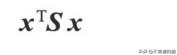在许多应用中，我们假设能量是正的，因此，相应的S应该是正定的。

测试正定性有许多等效条件。如果以下任何测试为真，则对称矩阵S为正定的：

1.所有特征值> 0，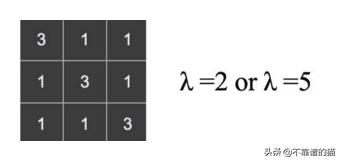2.所有左上角的行列式> 0，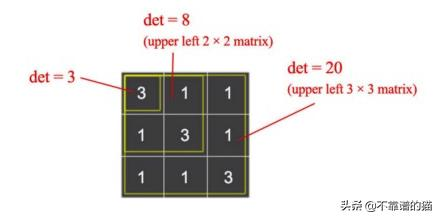3.所有pivots > 0，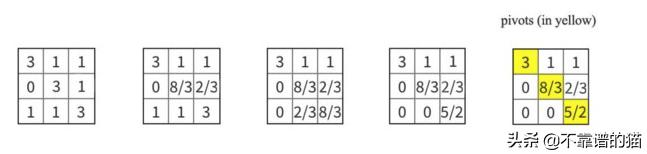4.能量(energy)> 0，除了x = 0，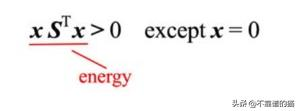5. S可以由一个列向量无关的矩阵a构成。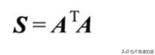验证所有特征值是正的需要很多工作。因此，条件2或3是更常见的测试。例如，正pivots 意味着正特征值(反之亦然)。另一方面，如果我们通过上述测试之一证明矩阵是正定的，我们保证它拥有上述所有属性。

证明

在本节中，我们将证明上面的一些属性。如果S是正定的，则​​所有λ都是正的。因此，相应状态x的计算能量为正(x = 0除外)。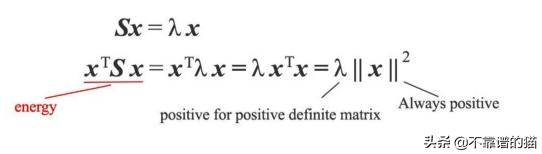如果S由AᵀA组成，则S在能量测试下为正。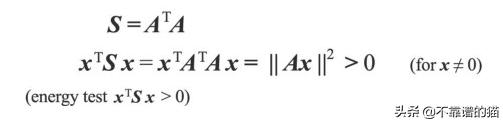除了正定，我们还有半正定，负定和半负定。半正定用“≥”替换上面的所有“>”条件(例如，它的特征值是大于或等于0 )，负定和半负定与正定和半正定相反。

Minimum

在微积分中，我们将f的一阶导数设置为零以找到其临界点。然而，这样的点可以是最大值，最小值或鞍点。许多机器学习模型以二次形式xAᵀx表示其成本函数。知道这个函数是否是凸函数是很重要的。因为如果它是凸的，我们知道局部最小值也是全局最小值。如果A是正定的，则​​二次函数是凸的。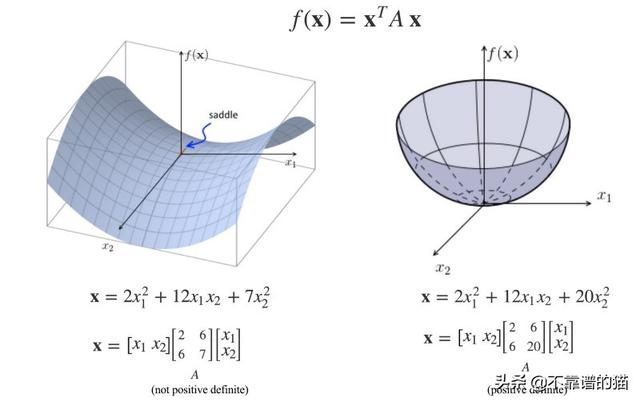对于任何函数f，我们计算下面的Hessian矩阵。如果A是正定的，则​​相应的点是局部最小值。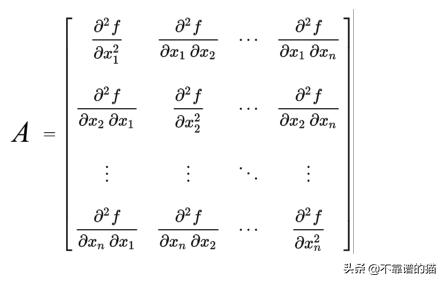协方差矩阵

在机器学习中，我们非常有兴趣找到特征之间的相关性。下图显示了重量和高度之间的正相关关系。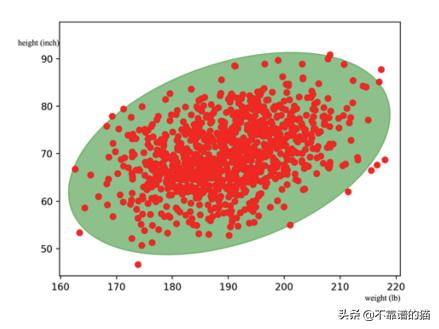在机器学习中，我们用协方差矩阵Σ建模关系。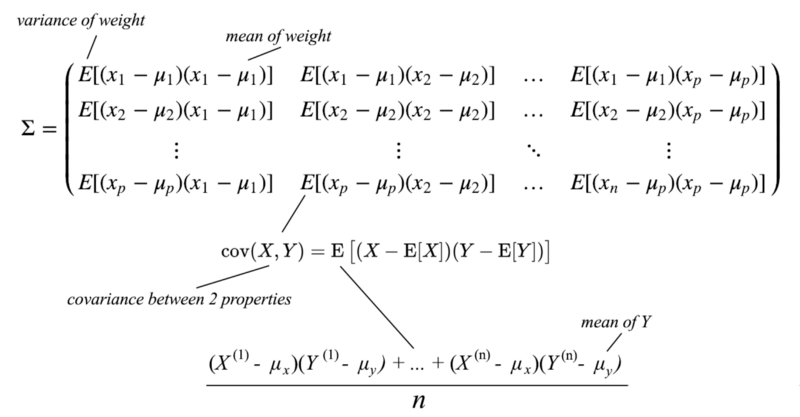协方差矩阵是半正定的。

展开全文weixin_39711441 2020-10-21 22:12:45
• %% 直线为轴中心对称的圆clc;clear;hold onsyms x y;% 画对称轴x1=-20:0.1:20;k=1;b1=10;f1(x)=k.*x+b1;plot(x1,f1(x1));theta=0:2*pi/3600:2*pi;R=4;Circle1=10+R*cos(theta);Circle2=10+R*sin(theta);plot(Circle1...

%% 直线为轴中心对称的圆

clc;

clear;

hold on

syms x y;

% 画对称轴

x1=-20:0.1:20;

k=1;

b1=10;

f1(x)=k.*x+b1;

plot(x1,f1(x1));

theta=0:2*pi/3600:2*pi;

R=4;

Circle1=10+R*cos(theta);

Circle2=10+R*sin(theta);

plot(Circle1,Circle2,'m','Linewidth',3);

x1=Circle1;

y1=Circle2;

% 对称点坐标(2*x0-x1,2f(x0)-y1)

b0=y1+(1/k).*x1;

x0=(b0-b1).*(k/(k^2+1));

x2=2*x0-x1;

y2=2*f1(x0)-y1;

plot(x2,y2,'r-');

axis equal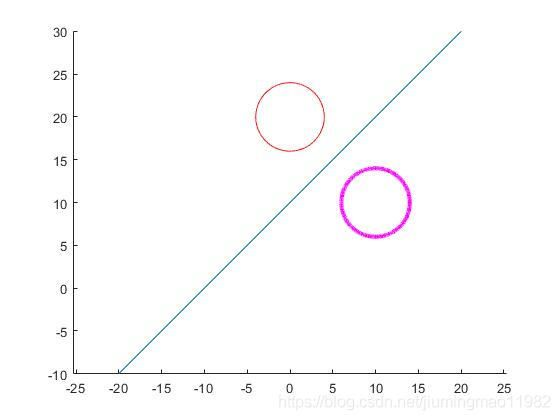%% 直线为轴中心对称的三角形

close all; clear all; clc

hold on

x1=-20:0.1:20;

syms x

k=1/2;

b1=4;

f1(x)=k.*(x+2)+b1;

plot(x1,f1(x1),'b');

x1=1;x2=2;x3=1;

y1=1;y2=2;y3=3;

triangle_x1=[x1,x2,x3,x1];

triangle_y1=[y1,y2,y3,y1];

fill(triangle_x1,triangle_y1,'r');

% 对称点坐标(2*x0-x1,2f(x0)-y1)

b0=triangle_y1+(1/k).* triangle_x1;

x0=(b0-b1).*(k/(k^2+1));

triangle_x2=2*x0-triangle_x1;

triangle_y2=2*f1(x0)-triangle_y1;

% triangle_y2=[1,2,1,1];

fill(triangle_x2,triangle_y2,'b');

axis([-5 5 -5 30])

axis equal;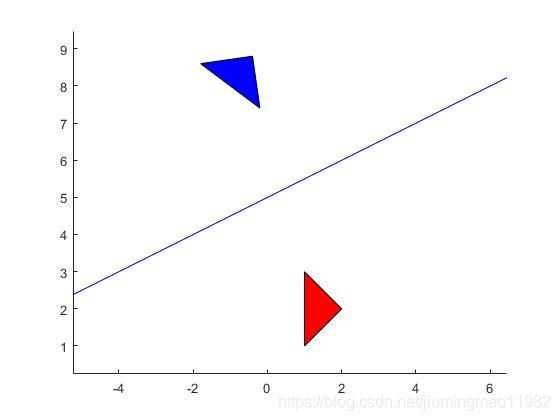%% 点为中心对称的三角形

close all; clear all; clc

hold on

x0=1.5;

y0=2;

plot(x0,y0,'*')

x1=1;x2=2;x3=1;

y1=1;y2=2;y3=3;

triangle_x1=[x1,x2,x3,x1];

triangle_y1=[y1,y2,y3,y1];

fill(triangle_x1,triangle_y1,'r');

triangle_x2=2*x0-triangle_x1;

triangle_y2=2*y0-triangle_y1;

fill(triangle_x2,triangle_y2,'b');

axis equal;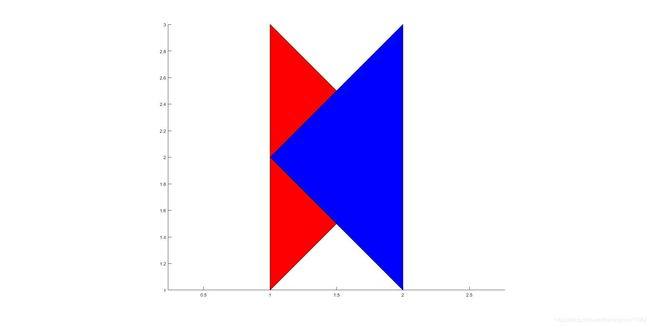展开全文weixin_39628342 2021-04-18 12:05:30
• 48KB weixin_38550334 2021-06-01 07:31:07
• 高中函数对称性总结 函数对称性常见公式

weixin_28737399 2020-12-24 11:10:10
• weixin_32736623 2021-05-25 06:36:55
• sitelist 2019-06-19 15:55:14
• 【LeetCode - 248】中心对称数 III leetcode java

qq_29051413 2020-09-26 17:05:51
• weixin_48673014 2021-07-15 20:32:47
• 判断中心对称 链表 中心对称

quxianpin 2014-03-31 17:57:30
• 链式表示的线性表之一——单链表4——判断字符串是否中心对称 数据结构与算法 单链表

baidu_36669549 2018-11-24 16:15:18
• AgoniAngel 2020-03-05 18:08:41
• 字符串处理 镜像对称 中心对称 字符串 对称

zjsunzone 2013-05-01 09:52:43
• 226KB weixin_38519849 2021-05-26 05:28:30
• 604KB weixin_38653691 2021-05-22 10:30:02
• weixin_34125314 2021-05-07 00:57:03
• 函数的对称性 函数对称性常见公式

weixin_42517044 2020-12-24 11:10:11
• 482KB weixin_38670186 2021-06-13 11:23:23
• 对称数的简单实现 对称数java算法

weixin_42513988 2021-03-09 23:36:37
• 60KB weixin_38704156 2021-05-26 23:27:49
• weixin_29149341 2021-01-17 17:38:48
• 对称加密、非对称加密、公钥、私钥究竟是个啥？ 加密 非对称加密 对称加密

shihengzhen101 2020-06-07 23:29:20
• 使用js找对称数 javascript

Y_web 2021-08-30 17:08:10
• 求一个半波对称振子天线的CST仿真 半波对称振子方向图

weixin_39969881 2020-12-19 06:32:09
• weixin_39631467 2021-03-09 09:24:33
• shangsongwww 2019-05-15 18:36:45
• weixin_29410963 2021-01-14 07:15:35...